# Problems With The Duology Of Computer Science Questions Posted By Aman Banka

PS: This note has been written to explain the problems with the question posted by a new user whose question context bewildered many people who tried to attempt the question but couldn't understand it, and also to explain the peculiar output of the program posted in the solution. This note is no way intended to insult the user, but rather to show him the mistakes that he did in framing the question and the program.

Recently, a newbie user Aman Banka posted a Computer Science question which had provisions of yielding infinitely many answers as such (the question was posted with the scope of a single answer only). Since the problem has been deleted now, we show you the screenshot of the question posted by him:So, it is pretty evident that the difference between the sums must depend on the diagonal sums, which in turn depend on the values entered in the diagonals of the square matrix. Clearly, the difference won't be a constant. As a result, user Rishabh Cool posted a report on the question, mentioning the same thing that the difference won't be constant as such.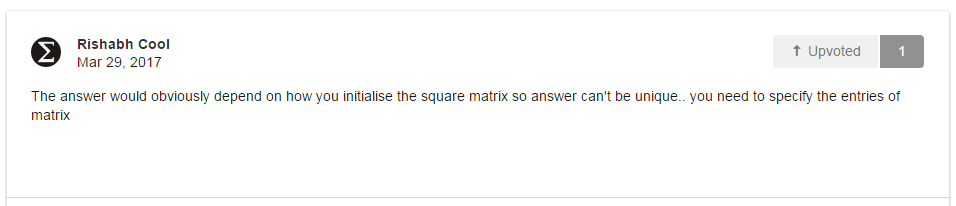But Aman Banka didn't provide a proper explanation and didn't understand that the answer won't be unique. The answer set to his question was 1, claiming that his program was showing the difference between the left and right diagonal sum of any matrix as 1. (Which obviously hints at a huge error in his program) But Aman Banka deletes this problem and then posts another problem which again sounds meaningless: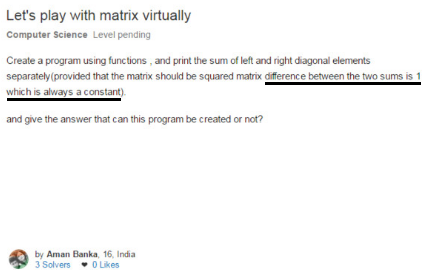difference between the two sums must be 1 which is always a constant

After reading this, the first question that must arise in someone's mind is that how can the difference between the sums be a constant if the matrix can be filled up in infinitely many ways, and it is the values which account for the difference between the diagonal sums. One solution (yet a stupid one) is to assign $s2 = s1 - 1$. However, the only thing that can be comprehended from here is that the question (supposedly) is asking that the right diagonal sum and left diagonal sum must be printed if and only if the difference between the sums is $1$, which can be easily be reached at through this:

  1 2 3 4 5 6 7 8 9 10 if(s2-s1==1 || s1-s2==1) //the sum will be shown if and only if the difference of the sums is 1 { cout<<"The difference between the left and right diagonal sum is 1, \nso the sum of the left diagonal elements and right diagonal sum will be printed.\n"; cout<<"Sum of the left diagonal elements = "<

 1 2 3 4 5 6 if (s1-s2 == 1) | (s2-s1 == 1): print("Difference is 1, so sums will be printed:") print("Left diagonal sum = ",s1) print("Right diagonal sum = ",s2) else: print("Difference is not 1, so sums will not be printed") 

Where $s1$ and $s2$ denote the left diagonal sum and the right diagonal sum respectively

But this is too far fetched. Finally, Calvin Lin posts a report on it, marking the deletion of this question because the question has not been phrased in a proper manner.But why was that program created by Aman Banka showing a constant difference of 1 everytime?

Well, why don't we take a look at the program that he posted?

  1 2 3 4 5 6 7 8 9 10 11 12 13 14 15 16 17 18 19 20 21 22 23 24 25 26 27 28 29 30 31 32 33 34 35 36 37 38 39 40 41 42 43 44 45 46 47 48 49 50 51 52 53 54 55 56 57 58 59 60 61 62 63 64 65 66 67 68 69 70 71 72 73 74 75 76 77 78 79 80 81 82 83 84 85 86 87 88 89 90 91 92 93 94 95 96 97 98 99 100 101 102 103 104 105 106 #include using namespace std; int matrix(int b,int row,int col); int main(){ int a,r,c; cout<<"enter rows and columns"<<"\n"; cin>>r; cin>>c; cout<<"enter the elements of matrix"<>a[i][j]; } } cout<<"\n"; cout<<"\n"; for(i=0;i0;f--){ for(g=c-1;g>0;g--){ if(f==g){ sum1=sum1+b[f][g]; } } } cout<<"\n"; cout<<"The sum of left diagonal element is "<

Check lines 87-97, there's where he had committed the mistake. His program aims at finding the left diagonal sum and right diagonal sum of a squared matrix. But the method of finding the sum of right diagonal sum is incorrect. The flow of control of a for-loop like this: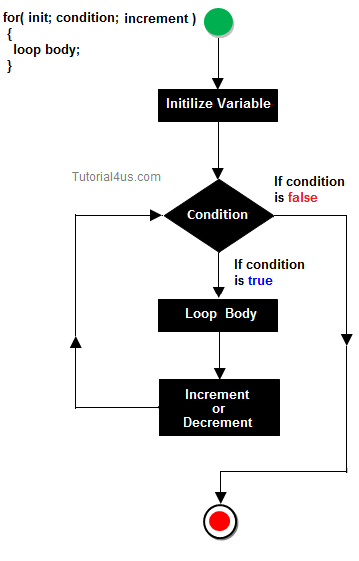And the loop considered here is:

  1 2 3 4 5 6 7 8 9 10 11 12 for(f=r-1;f>0;f--){ for(g=c-1;g>0;g--){ if(f==g){ sum1=sum1+b[f][g]; } 

So, this loop actually sums up the elements of the left diagonal only, but except the $$ element, because, the condition given is that the row index and column index must be more than 0. So the last element to be summed is $$ since $1>0$ but in the next step, it decreases to 0, which marks the end of the loop as 0 is not more than 0 and doesn't satisfy the condition of the loop anymore.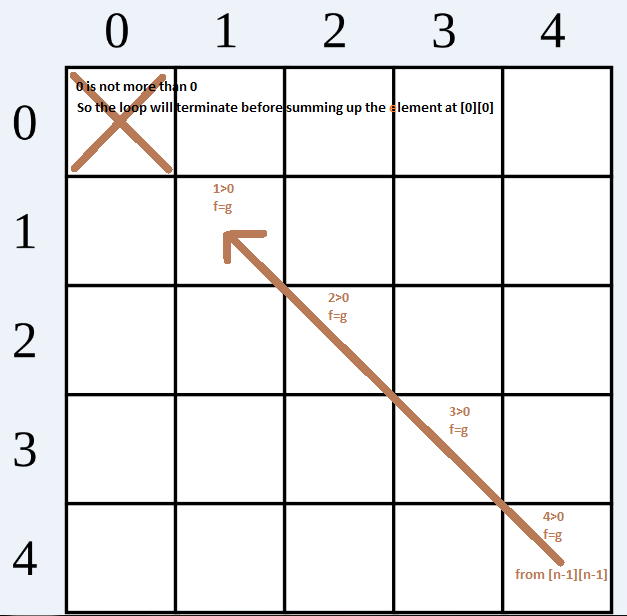Since the right diagonal sum here is the left diagonal sum only, but without the $$ element being added to it, the difference between the left diagonal sum and the right diagonal sum will always be equal to the value entered at $$. In Aman Banka's output, he had entered 1 in $$ and as expected his difference came out to be 1.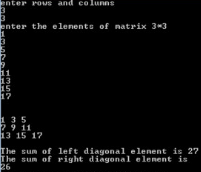In a nutshell, the difference of the sums in this program will always be equal to the value entered at $$, and is irrespective of the other values entered in the square matrix. For example, if you enter 'x' at $$ and fill up other elements in the array with any number you wish, you will still find that the difference between the sums is equal to 'x'.

Then, what should be the rectification?

The right diagonal of a square matrix is actually this: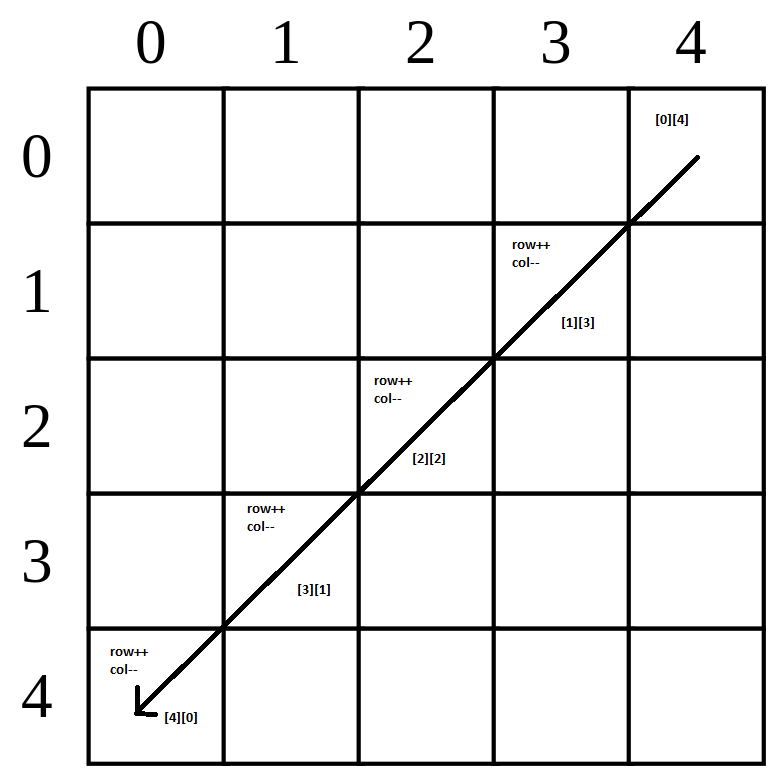As we see for an (n by n) matrix, we have to start adding from $[n-1]$, and each succeeding right diagonal element has row index $1$ more than the previous row index and has column index $1$ less than the previous column index, which must run till the row index becomes equal to $n-1$, i.e, till it is lesser than $n$. So, we can grab the intuition from here that the loop must be like this:

 1 2 3 4 5 6 j=n-1; //the first right diagonal element has column index n-1 for(i=0;i

Where, $i$ and $j$ are the row and column index counter and $s2$ is the sum of the right diagonal elements initiated at 0.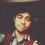Note by Arkajyoti Banerjee
3 years, 3 months ago

This discussion board is a place to discuss our Daily Challenges and the math and science related to those challenges. Explanations are more than just a solution — they should explain the steps and thinking strategies that you used to obtain the solution. Comments should further the discussion of math and science.

When posting on Brilliant:

• Use the emojis to react to an explanation, whether you're congratulating a job well done , or just really confused .
• Ask specific questions about the challenge or the steps in somebody's explanation. Well-posed questions can add a lot to the discussion, but posting "I don't understand!" doesn't help anyone.
• Try to contribute something new to the discussion, whether it is an extension, generalization or other idea related to the challenge.

MarkdownAppears as
*italics* or _italics_ italics
**bold** or __bold__ bold
- bulleted- list
• bulleted
• list
1. numbered2. list
1. numbered
2. list
Note: you must add a full line of space before and after lists for them to show up correctly
paragraph 1paragraph 2

paragraph 1

paragraph 2

[example link](https://brilliant.org)example link
> This is a quote
This is a quote
    # I indented these lines
# 4 spaces, and now they show
# up as a code block.

print "hello world"
# I indented these lines
# 4 spaces, and now they show
# up as a code block.

print "hello world"
MathAppears as
Remember to wrap math in $$ ... $$ or $ ... $ to ensure proper formatting.
2 \times 3 $2 \times 3$
2^{34} $2^{34}$
a_{i-1} $a_{i-1}$
\frac{2}{3} $\frac{2}{3}$
\sqrt{2} $\sqrt{2}$
\sum_{i=1}^3 $\sum_{i=1}^3$
\sin \theta $\sin \theta$
\boxed{123} $\boxed{123}$

Sort by:

for second diagonal we could also use i+j=n condition

- 3 years, 2 months ago

You happen to mean $i + j = n - 1$, right? Array indices start from $0$ to $n-1$.

Your approach is certainly aiming at:

 1 2 3 4 for(int i = 0; i < n; i++) for(int j = 0; j < n; j++) if(i + j == n - 1) s += a[i][j]; 

This would work, undoubtedly. But note that this block of code will run through a number of $n^2$ iterations, making it slower than that of mine involving just $n$ iterations.

- 3 years, 2 months ago

What is meant by duology here?

- 3 years, 3 months ago

A set or compilation of 2 things. (Duo-logy)

- 3 years, 3 months ago

Nice... This might clarify his misunderstanding..

- 3 years, 3 months ago

I hope it does :3

- 3 years, 3 months ago